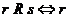Chapter 8.5, Problem 6ES### Discrete Mathematics With Applicat...

5th Edition
EPP + 1 other
ISBN: 9781337694193

#### Solutions

Chapter
Section### Discrete Mathematics With Applicat...

5th Edition
EPP + 1 other
ISBN: 9781337694193
Textbook Problem
1 views

# Let P be the set of all people who have ever lived and define a relation R on P as follows: For every r ,   s ∈ P ,is an ancestor of s or r = s . Is R a partial order relation? Prove or give a counterexample.

To determine

To prove or give the counter example for R to be a partial order relation.

Explanation

Given information:

Let P be the set of all people who have ever lived and define a relation R on P as follows: for all r,sP, rRs r is an ancestor of s or r=s.

Calculation:

A partial order relation is a relation which is reflexive, antisymmetric and transitive.

So let us verify the following all three properties for R to be a partial order relation.

Reflexivity- Suppose rPrRr because r=r. Therefore the relation R is reflexive.

Antisymmetry- Suppose r,sP, such that rRs r is an ancestor of s or r=s....(1).

And sRr s is an ancestor of r or s=r....(2)

### Still sussing out bartleby?

Check out a sample textbook solution.

See a sample solution

#### The Solution to Your Study Problems

Bartleby provides explanations to thousands of textbook problems written by our experts, many with advanced degrees!

Get Started